Next: Perihelion Precession of Mercury Up: Gravitational Potential Theory Previous: Potential Due to a

# Perihelion Precession of the Planets

The Solar System consists of eight major planets (Mercury to Neptune) moving around the Sun in slightly elliptical orbits which are approximately co-planar with one another. According to Chapter 5, if we neglect the relatively weak interplanetary gravitational interactions then the perihelia of the various planets (i.e., the points on their orbits at which they are closest to the Sun) remain fixed in space. However, once these interactions are taken into account, it turns out that the planetary perihelia all slowly precess around the Sun. We can calculate the approximate rate of perihelion precession of a given planet by treating the other planets as uniform concentric rings, centered on the Sun, of mass equal to the planetary mass, and radius equal to the mean orbital radius.This is equivalent to averaging the interplanetary gravitational interactions over the orbits of the other planets. It is reasonable to do this, since the precession period in question is very much longer than the orbital period of any planet in the Solar System. Thus, by treating the other planets as rings, we can calculate the mean gravitational perturbation due to these planets, and, thereby, determine the desired precession rate.

We can conveniently index the planets in the Solar System such that Mercury is planet 1, and Neptune planet 8. Let the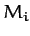and the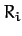, for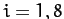, be the planetary masses and orbital radii, respectively. Furthermore, let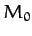be the mass of the Sun. It follows, from the previous section, that the gravitational potential generated at the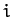th planet by the Sun and the other planets is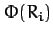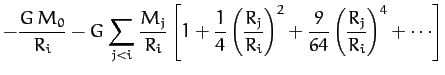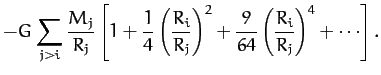(1018)

Now, the radial force per unit mass acting on theth planet is written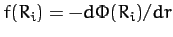, giving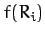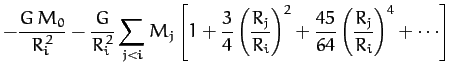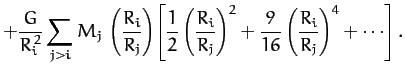(1019)

Hence, we obtain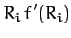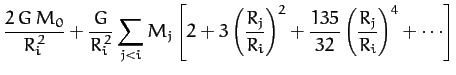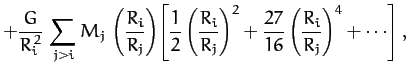(1020)

where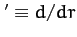. It follows that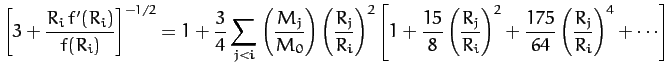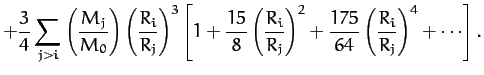(1021)

Thus, according to Equation (317), the apsidal angle for theth planet is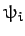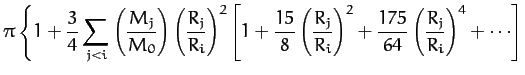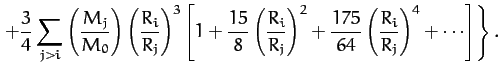(1022)

Hence, the perihelion of theth planet advances by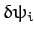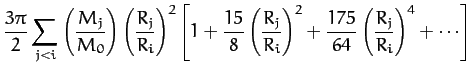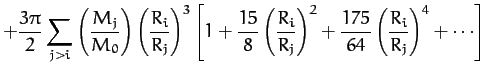(1023)

radians per revolution around the Sun. Now, the time for one revolution is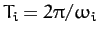, where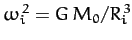. Thus, the rate of perihelion precession, in arc seconds per year, is given by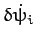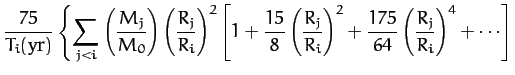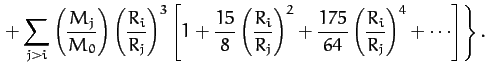(1024)

Table 1: Data for the major planets in the Solar System, giving the planetary mass relative to that of the Sun, the orbital period in years, and the mean orbital radius relative to that of the Earth.
 Planet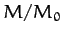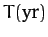R( au) Mercury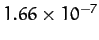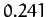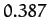Venus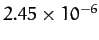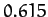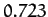Earth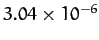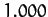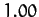Mars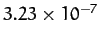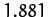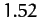Jupiter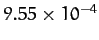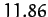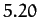Saturn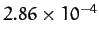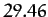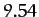Uranus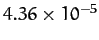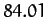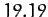Neptune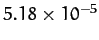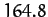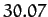Table 2: The observed perihelion precession rates of the planets compared with the theoretical precession rates calculated from Equation (1024) and Table 1. The precession rates are in arc seconds per year.
 Planet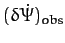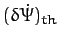Mercury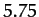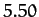Venus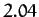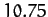Earth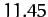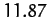Mars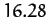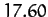Jupiter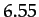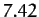Saturn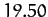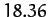Uranus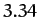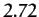Neptune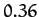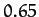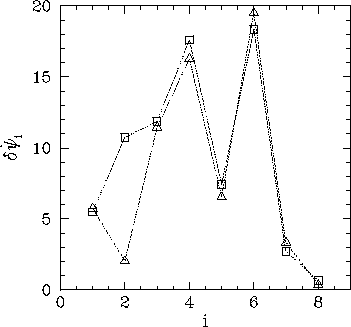Table 2 and Figure 46 compare the observed perihelion precession rates with the theoretical rates calculated from Equation (1024) and the planetary data given in Table 1. It can be seen that there is excellent agreement between the two, except for the planet Venus. The main reason for this is that Venus has an unusually low eccentricity (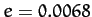), which renders its perihelion point extremely sensitive to small perturbations.Next: Perihelion Precession of Mercury Up: Gravitational Potential Theory Previous: Potential Due to a
Richard Fitzpatrick 2011-03-31